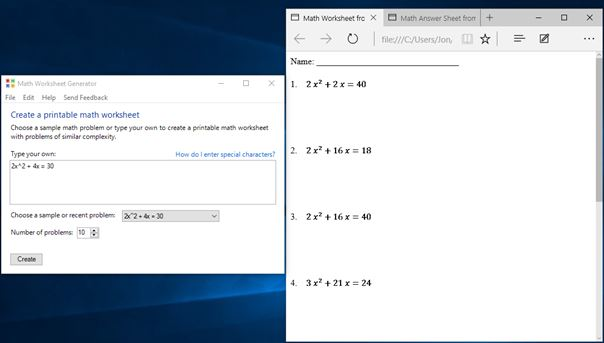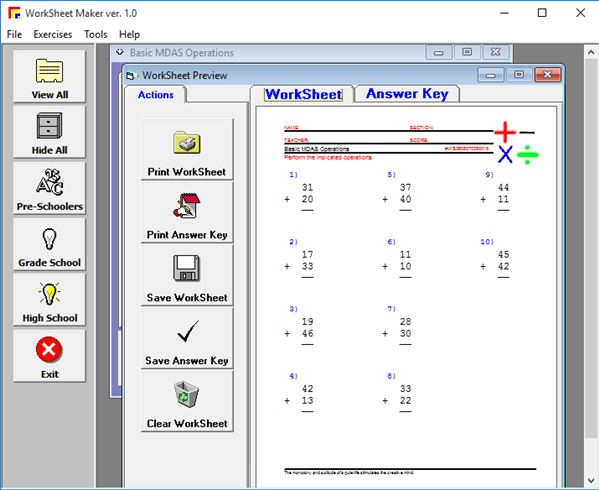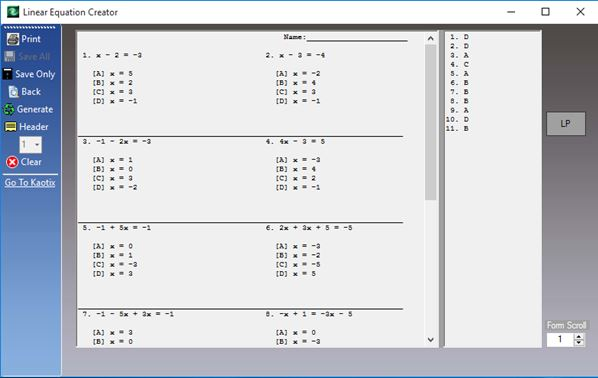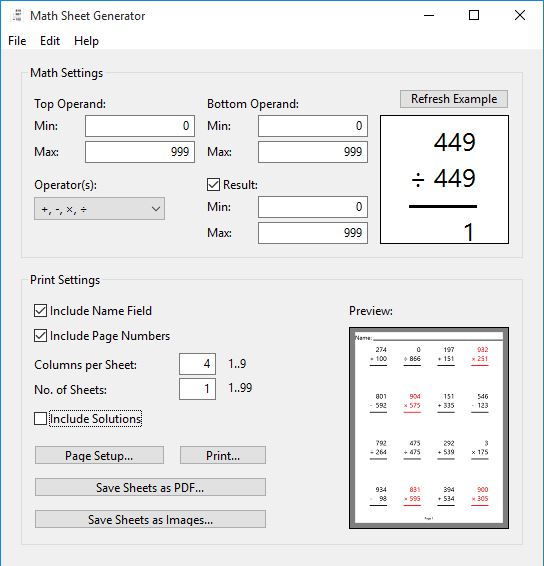Editor Ratings:
User Ratings:
[Total: 2 Average: 3]

Here’s a list of 4 math worksheet generator software for Windows 10. They can be used to create worksheets with math problems on your Windows 10 powered devices. When it comes to math the old expression “practice makes perfect” still holds water today. Summer’s here, and not a lot of learning will be done during it. However if you have a kid that’s very interested in math, or one that has summer school in math, then applications from the list down below are just the thing you need.

Lets have a look at what exactly it is that’s available.

## Microsoft Math Worksheet GeneratorMicrosoft Math Worksheet Generator is a very simplistic math worksheet generator software for Windows 10 which can be used to create a large number of different math problems, ranging from basics like addition, subtraction, multiplication, division, to the more advanced ones like equations with two variables (x and y). After launching Math Worksheet Generator, you’ll see a simplistic interface (see image above).

Two things have to be setup in order for the worksheet to be generated. First the math problem sample needs to be set. On the image above I’m using an equation with a single variable. There are other presets for addition, multiplication and everything else that I already mentioned. Second thing that you need to tweak is the number of math problems that are going to be generated. How it basically works is by using the sample math problem that you enter in order to create similar ones. They are generated in a PDF document alongside an answer key where the correct results for the generated math problems can be found. There is a syntax that you need to follow when writing math samples. You can read up on it by opening up the help pages.

Get Microsoft Math Worksheet Generator here.

## iKodeko Worksheet MakeriKodeko Worksheet Maker is an advanced worksheet generator software for Windows 10. It can be used to create worksheets for pre-schoolers, grade school students and high school students. Use the left sidebar in the main application window to choose for which of the three available levels of education you need the worksheets for.

Selecting one will open up additional windows on the right. Here you can’t write math problem samples of your own. Instead you choose them from the available presets. Presets include the basics like addition, subtraction, division, multiplication and the more advanced stuff like fractions, equations, Pythagorean theorem, number systems and more. Worksheet is created by clicking on the “Generate” button. Preview is given for both the worksheet with the math problems and the answer key, where solutions are given. Generated worksheets and answer keys can be printed or saved as PDFs.

Also, have a look at 9 Free Websites to Learn Maths for Kids.

## Linear Equation Generator – free math worksheet generator software for Windows 10Linear Equation Generator is a math worksheet generator software for Windows 10 which focuses on creating equation based math problems. 5 different types of linear equations can be created. They can be fully configured including the number of solutions (one, none, infinity), types of solutions (integers, fractions), number of variables and more.

How many equations per page, and how pages are going to be generated is also customizable. This means that you can for example generate 10 pages with 20 equations per page at once. When everything is setup you’ll see a “Preview” button to preview how the equations are going going to look like, see image above. If everything’s OK, click either the “Save only” button to save worksheet as a PDF document or “Print” to print it to paper directly from the application.

## MathSheetGeneratorMathSheetGenerator is a another simplistic math worksheet generator software for Windows 10 which can be used to generate worksheets for basic algebra only. Worksheets can be extremely customized, as you can see from the image above.

How the worksheet is going to look like is highly customizable, as well as the math problems themselves. Number of problems per page, and the number of pages that are going to be generated can be controlled using the “Print” settings” area at the bottom of the application window. “Math settings” section holds options for configuring how high the numbers will go in the generated math problems, and which algebra operations are going to be used. “Refresh Example” button in the top right corner is going to change the worksheet preview in the bottom right corner. When done, worksheet can be printed directly via printer, or saved as either PDF document or images.

Also, have a look at Create Online Math Worksheets And Share, Publish Them Using MathDisk.

## Conclusion

All 4 of the math worksheet generator software for Windows 10 from the list above worked great. iKodeko Worksheet Maker seems like the best choice if you are a teacher and you need to generate worksheets with lots of different types of math problems. If you need something a bit more specific, like linear equations, go with Linear Equation Generator. Let me know what you think by leaving a comment down below.

 Editor Ratings: User Ratings:[Total: 2 Average: 3] Works With: Windows 10 Free/Paid: Free Tags: Windows 10